# Pandas DataFrame

It is a data structure used to represent data into the row and column format (tabular format). It can store similar or different types of data. Pandas provides DataFrame with lots of attributes and methods that are used to perform various operations like transpose, copy, append, trim, etc.

In other words, we can say that DataFrame is a two-dimensional data structure that has a mutable size, and allows us to store heterogeneous data.

A sample example of DataFrame is shown in the below image.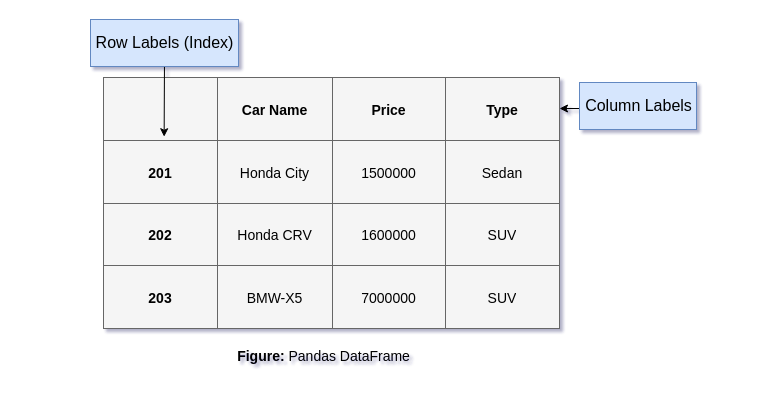After knowing to DataFrame, Now the point is how to create dataframe?To create DataFrame, Pandas Provides DataFrame constructor that helps to generate DataFrame from various data models likelist, dict, NumPy array or CSV file, etc.

## Pandas DataFrame Constructor Syntax

The following is the syntax of the DataFrame constructor. We can use it to get DataFrame from dict, list, CSV file, etc.

``````DataFrame(data=None, index: Optional[Collection] = None,
columns: Optional[Collection] = None,
dtype: Optional[Union[str, numpy.dtype, ExtensionDtype]] = None,
copy: bool = False
)``````

## DataFrame Constructor's Parameters

 Parameter Name Description data Data can be an Iterable, dict, ndarray, or DataFrame. index It sets a row index for DataFrame. If not provided, default to RangeIndex. columns It is used to set column labels for the resulting frame. If not provided, will default to RangeIndex (0, 1, 2, …, n). dtype It is used to set the data type explicitly. Only a single dtype is allowed. If no datatype is provided, it infers from data. copy It is used to copy data from inputs.

## How to create DataFrame in Pandas?

There may be several ways to create DataFrame but here we are using DataFrame's constructor. The following are the type of data that we can pass to DataFrame's constructor to get DataFrame.

• Numpy
• Dictionary
• List
• File

## Creating DataFrame from Numpy Array

In this example, we are using the NumPy library to create an array of elements and after that passing that array to get DataFrame. It is the simplest and easy way to convert Numpy array to DataFrame.

``````import pandas as pd
import numpy as np
# Create Array using Numpy
data = np.array([[1.0, 1.1], [2.0, 2.1], [3.0, 3.1]])
# Convert numpy array to DataFrame
df = pd.DataFrame(data)
print(df)``````

Output: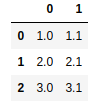## Jupyter Notebook

If you are familiar with Jupyter and using Jupyter notebook to practice the Pandas then the above example can be executed like below.

If you are a beginner and not aware of Jupyter notebook then we recommend you skip this step and scroll down to read further.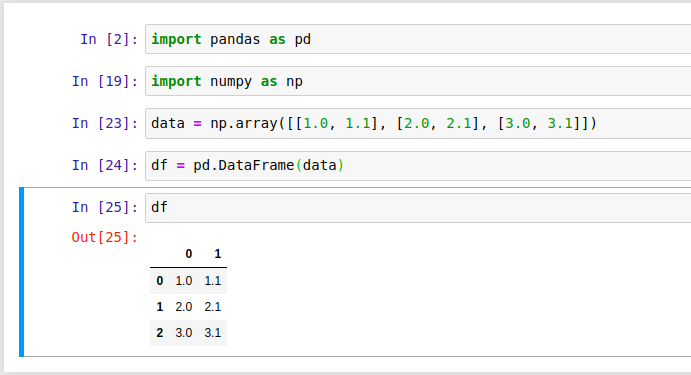## Creating DataFrame from List

In this example, we are using a list to create a DataFrame. The list contains several lists that represent 2D array and then converting the list to DataFrame using DataFrame's constructor.

``````import pandas as pd
# Take a list of lists
data = [[1.0, 1.1], [2.0, 2.1], [3.0, 3.1]]
# Convert list to DataFrame
df = pd.DataFrame(data)
print(df)``````

Output:## Creating DataFrame from Dictionary in pandas

In this example, we are creating DataFrame by using a dictionary data model. The dictionary is passed to the DataFrame's constructor to get DataFrame from the dictionary.

``````import pandas as pd
# Take a Dictionary
data = {1:[1.0, 1.1], 2:[2.0, 2.1], 3:[3.0, 3.1]}
# Creating Dictionary to DataFrame
df = pd.DataFrame(data)
print(df)``````

Output: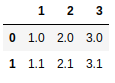## How to create DataFrame from CSV File?

If we want to create a DataFrame from a CSV then we can use the Pandas read_csv() method that takes a file as an argument and returns a DataFrame as a result. For example, we have a file data.csv that contains data. The file is shown below.

// File: data.csv

``````Car Name, Price
Maruti Brezza, 1000000
BMW X5, 6000000
Honda CRV, 1500000
Duster, 1500000``````

## Example: Create DataFrame from File

In this example, we are using the read_csv() method to read the data.csv file's data. See, as a result, it returns DataFrame and the first line of the file (data.csv) is converted to DataFrame's columns labels. See the example below.

``````import pandas as pd
df = pd.read_csv("data.csv") # getting DataFrame
print(df)``````

Output:## Rename Columns and Index of DataFrame in pandas

In our previous examples, we created DataFrame in several ways like list, dictionary, etc. Notice that columns and index (row) labels are set to default values. If we wish to rename them, we can set new values for columns and index (row) of the DataFrame as explained in the below example.

``````import pandas as pd
# Take a list of lists
data = [[1.0, 1.1], [2.0, 2.1], [3.0, 3.1]]
# Create dataframe from the list
df = pd.DataFrame(data)
# Setting new columns labels
df.columns = ['A','B','C']
# Setting index (row) labels
df.index = ['x','y']
print(df)
``````

Output: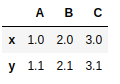### Conclusion

Well, in this topic, we have learned to create DataFrame by using DataFrame's constructor with several data models like Python dictionary, list, NumPy array and CSV file. We explained the topic with the help of several examples.

If we missed something, you can suggest us at - info.javaexercise@gmail.com

Trending